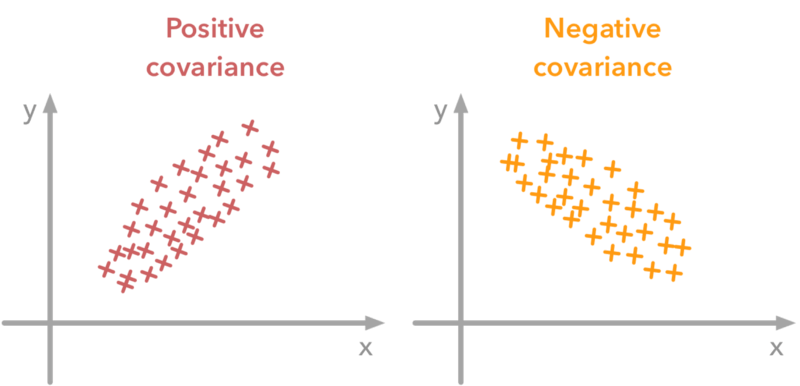# Covariance Calculator

Enter the values for dataset X and Y in the given input boxes. Hit that Calculate button to get the covariance and mean value for X and Y using sample covariance calculator.## Covariance formula

The following covariance equation is the sample covariance formula Cov(x,y) if two sample sizes are available.

Covsam (x, y) = sum (xi – x mean) (yi – y mean) / n

Where:

mean refers to the mean value of these sample elements.

meanrefers to the mean value of second sample elements.

xi– x mean represents the difference between sample elements for X and the mean value of the sample.

yi– y mean represents the difference between sample elements for and the mean value of the sample.

n represents the size of the sample for both X and Y.

The covariance of x and y calculator above uses this equation to find the covariance.

Covariance calculator is an online statistical tool that is used to calculate the relationship between the two sets of variables X and Y that are commonly described.

It also calculates the mean value for X and Y along with calculating covariance. Here, we will explain covariance definition, how to find covariance, formula for covariance, and how do you calculate covariance with examples.

## What is covariance?

Covariance measures how many random (X, Y) variables in each population are distinct. When the population is greater or random, the matrix represents the relation between different dimensions.

Variance, covariance, and coefficient of variance are vitally associated to each other.

### Properties of covariance

Covariance properties can be stated as:

• The smaller Xand greater Y values give the positive covariance.

X < Y è +ve covariance

• The greater Xand the smaller Y values shows covariance is negative.

X > Y è -ve covariance

• The covariance would be negative or non-linear if all random variables are not statistically dependent.## How to calculate covariance?

Calculating covariance is comparatively easy if you use stock covariance calculator. Manual calculation is bit tricky but we will explain the method by keeping it as simple as possible.

Example:

Betty has purchased the stocks of Retro plastics recently. She wants to purchase one more company’s stocks to broaden her investment but in different industry. She has got two great options after some research, i.e., sun autos and DM textiles. But she doesn’t know which option she should opt out.

She can decide by calculating the covariance for return on both houses.

Betty gets the five closing prices for both of the companies, sun autos and DM textiles.

Prices of sun autos represents xi, and prices of DM textiles represents yi.

 i xi yi 1 8.47 10.63 2 11.22 9.21 3 11.99 10.71 4 11.45 8.01 5 10.92 5.03 Mean value 10.81 8.718

Follow these steps carefully to get the covariance:

Step 1: Calculate the mean value for xi. Add all values and divide the sum by size of the sample (5).

mean = 10.81

Step 2: Calculate the mean value for ytoo. Add all values and divide the sum by size of the sample (5).

mean = 8.718

Step 3: Calculate the diff. Difference of X can be calculated by subtracting each element of x from mean.

diff = xi – x mean

To find differences for all x values, use the above equation and place them in a column.

Step 4: Repeat the previous step for y. Calculate ydiff by subtracting all values of y from the mean.

diff = yi – y mean

Step 5: Multiply all values of x diff and y diff and place them in the next column.

Step 6: Add the values of last column, which are the product of the two differences. After adding, divide the sum by the sample size. After dividing the sum by sample size, the resulting value will be the covariance.

Sum of differences = -1.65911

Cov sam (x, y) = -1.65911 / 5

Cov sam (x, y) = -0.3318

 i xi yi x diff y diff x diff × y diff 1 8.47 10.63 -2.34 1.912 -4.47 2 11.22 9.21 -0.144 0.958 -0.1380 3 11.99 10.71 0.626 2.458 1.5387 4 11.45 8.01 0.086 -0.242 -0.02081 5 10.92 5.03 -0.444 -3.222 1.431 Mean value 10.81 8.718 -1.65911

The covariance value -0.3318 gives us the idea about variation in the prices of both companies. By using this value, Betty can decide to buy stocks of one of them.

You can use the population covariance calculator above to cross-check the result of covariance calculation.